Open in App
Not now

# Shell Scripting – Test Command

• Difficulty Level : Easy
• Last Updated : 21 Feb, 2023

A test command is a command that is used to test the validity of a command. It checks whether the command/expression is true or false. It is used to check the type of file and the permissions related to a file. Test command returns 0 as a successful exit status if the command/expression is true, and returns 1 if the command/expression is false.

Syntax:

`test [expression]`

Example:

`test "variable1' operator "variable2"`

Here, expression can be any command or expression that can be evaluated by the shell. And it is recommended to always enclose out test variables into double-quotes.

Here, are some of the operator flags that can be used with test command, along with their meaning:

Flags for files and directories:

• test -e filename: Checks whether the file exists or not. And return 1 if file exists and returns 0 if file does not exist.
• test -d filename: Checks whether the file is a directory or not. And returns 0 if the file is a directory and returns 1 if the file is not a directory.
• test -f filename: Checks whether the file is a regular file or not. And returns 0 if the file is a regular file and returns 1 if the file is not a regular file.
• test -s filename: Checks whether the file is empty or not. And returns 0 if the file is not empty and returns 1 if the file is empty.
• test -r filename: Checks whether the file is readable or not. And returns 0 if the file is readable and returns 1 if the file is not readable.
• test -w filename: Checks whether the file is writable or not. And returns 0 if the file is writable and returns 1 if the file is not writable.
• test -x filename: Checks whether the file is executable or not. And returns 0 if the file is executable and returns 1 if the file is not executable.

Flags for  text strings

• string1 = string2: Checks whether the two strings are equal or not. And returns 0 if the two strings are equal and returns 1 if the two strings are not equal.
• string1 != string2: Checks whether the two strings are not equal or not. And returns 0 if the two strings are not equal and returns 1 if the two strings are equal.
• -n string: Checks whether the string is empty or not. And returns 1 if the string is empty and returns 0 if the string is not empty.
• -z string: Checks whether the string is empty or not. And returns 0 if the string is empty and returns 1 if the string is not empty.

Flags for comparison of numbers

• num1 -eq num2: Checks whether the two numbers are equal or not. And returns 0 if the two numbers are equal and returns 1 if the two numbers are not equal.
• num1 -ne num2: Checks whether the two numbers are not equal or not. And returns 0 if the two numbers are not equal and returns 1 if the two numbers are equal.
• num1 -gt num2: Checks whether the first number is greater than the second number or not. And returns 0 if the first number is greater than the second number and returns 1 if the first number is not greater than the second number.
• num1 -ge num2: Checks whether the first number is greater than or equal to the second number or not. And returns 0 if the first number is greater than or equal to the second number and returns 1 if the first number is not greater than or equal to the second number.
• num1 -lt num2: Checks whether the first number is less than the second number or not. And returns 0 if the first number is less than the second number and returns 1 if the first number is not less than the second number.
• num1 -le num2: Checks whether the first number is less than or equal to the second number or not. And returns 0 if the first number is less than or equal to the second number and returns 1 if the first number is not less than or equal to the second number.

Conditional flags

• condition1 -a condition2: Checks whether the two conditions are true or not. And returns 0 if both the conditions are true and returns 1 if either of the conditions are false.
• condition1 -o condition2: Checks whether the two conditions are true or not. And returns 0 if either of the conditions are true and returns 1 if both the conditions are false.
• !expression: Checks whether the expression is true or not. And returns 0 if the expression is false and returns 1 if the expression is true.

So let’s take a few examples to understand the test command better.

1. Numeric comparisons

Below is an example to check if two numbers are equal or not.

```#!/bin/bash
# Example to check if two numbers are equal
# or not

# first number
a=20

# second number
b=20

# using test command to check if numbers
# are equal
if test "\$a" -eq "\$b"
then
echo "a is equal to b"
else
echo "a is not equal to b"
fi```

Output: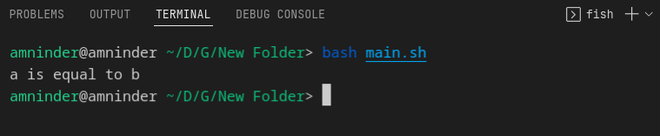Numeric comparison

2. String comparisons

Test command allows us to compare strings as well. We can check if two strings are equal or not, if one string is greater than the other if one string is less than the other, etc. Based on the string size, we can perform the comparison.

3. String equality

Below is a simple example to check the equality of two strings using the test command

```#!/bin/bash
# Example to check if two strings are equal or not
# first string
a="Hello"
b="Hello"
if test "\$a" = "\$b"
then
echo "a is equal to b"
else
echo "a is not equal to b"
fi```

Output:String equality comparison

4. String order

String order basically means checking if one string is greater than the other or not. Below is the example to check if one string is greater than the other.

Script:

```#!/bin/bash
# Example to check if one string is greater than
# the other
# first string
a="Hello"
b="Geek"
if test "\$a" \> "\$b"
then
echo "\$a is greater than \$b"
else
echo "\$a is not greater than \$b"
fi```

Output: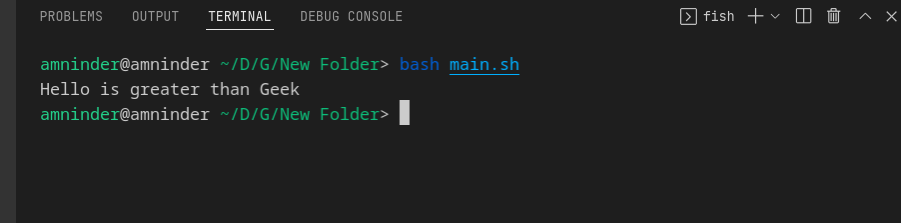String order comparison

We have to use ‘\>’ instead of ‘>’ because ‘>’ is a redirection operator. And to make it work, we have to use ‘\>’ instead of ‘>’. ‘\’ is an escape character.

5. String size

We can also compare strings based on their size using the test command. Below are some examples to perform the comparisons.

```#!/bin/bash
# Example to check if string contains some data
# or not

# first string
a="Hello"

# second string
b=""

# using test command on first string to check if
# string is empty or not
# -n will return true if string is not empty
if test -n "\$a"
then
echo "String a is not empty"
else
echo "String a is empty"
fi

# -z will return  true if string is empty
if test -z "\$b"
then
echo "String b is empty"
else
echo "String b is not empty"
fi```

Output: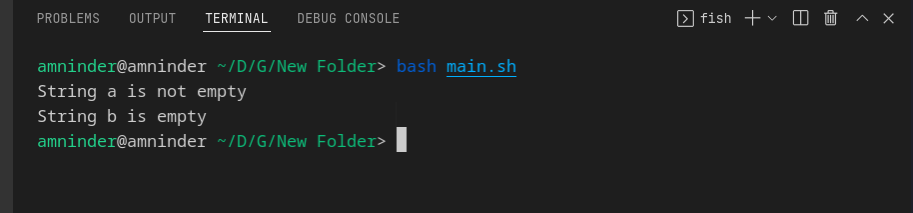String size comparison

6. File comparisons

Test command also aids us to test the status of files and folders on the Linux file system.  Below is an example to check if a file is empty or not. For this purpose, we can use the -s flag which will return true if the file size is greater than 0 means if the file is not empty and it returns false if the file is empty.

```#!/bin/bash
# Example to check if file is empty or not
# test command with -s flag to check if file
# is empty or not
# creating new file with the given name
filename="I_LOVE_GEEKSFORGEEKS.txt"

# touch command is used to create empty file
touch \$filename

# checking if file is empty or not
if test -s \$filename
then
echo "File is not empty"
else
echo "File is empty"
fi

# adding to the newly created text file
echo "I love GeeksforGeeks" >> \$filename

# checking again if file is empty or not
if test -s \$filename
then
echo "File is not empty now"
else
echo "File is empty"

fi```

Output: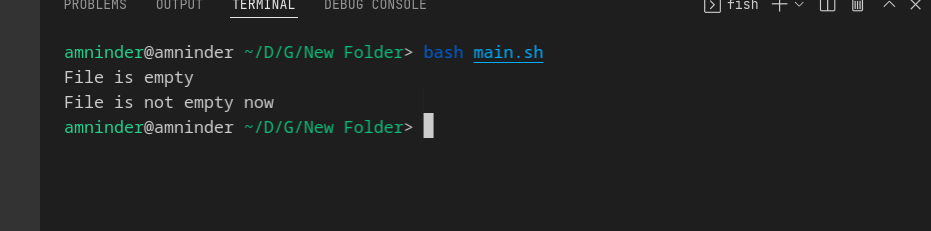file comparisons

Example :

Script to check if the script is running as root or not, using the test command.

```# check if the script is run as root or not
if test "\$(id -u)" == "0"
then
echo "This script is running as root"
else
echo "This script is not running as root"1>&2
exit 1

fi```

Output: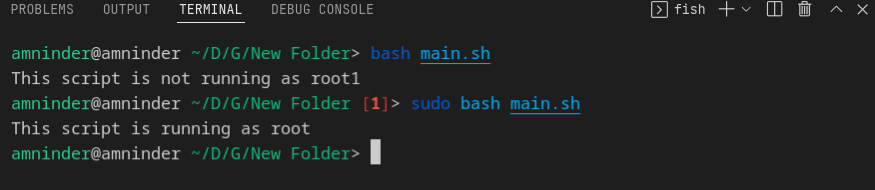Script to check if the script is running as root or not using the test command

Here, the -id u flag is used to check the user id of the user who is running the script. And if the user id is not 0 that means the user is not root and the script will print the else statement. The 1>&2 is used to redirect the output to stderr. Exit 1 will exit the script with a status code of 1 i.e., failure and if we do not use exit 1 the script will exit with a status code of 0 i.e., success.

Note: Here, the highlighted 1 in red color is used to indicate that the script that we run earlier was exit with status code 1.  Because it was not running as root.

My Personal Notes arrow_drop_up
Related Articles